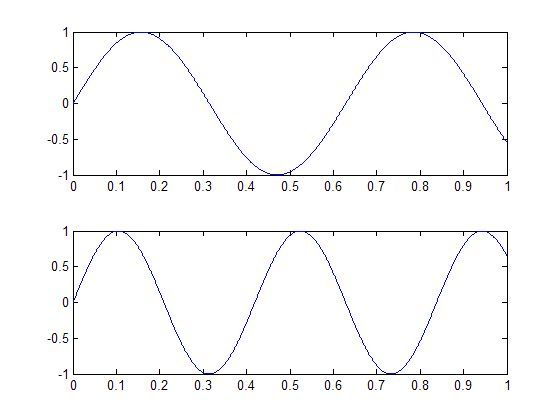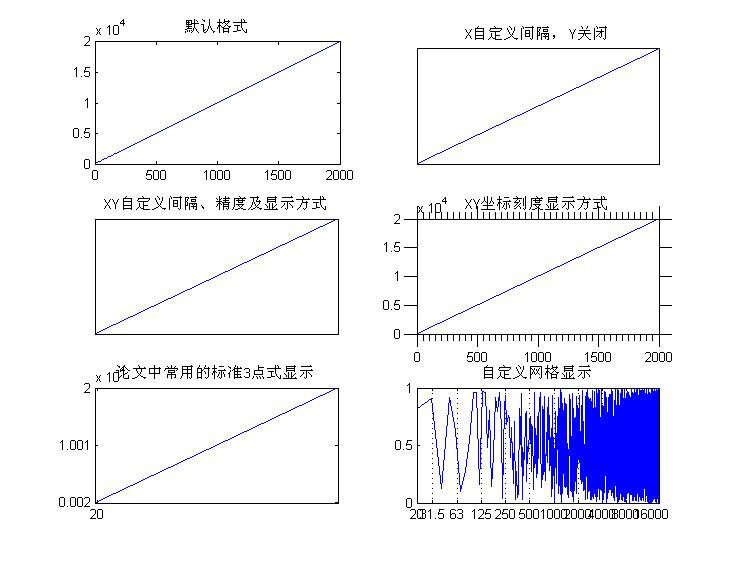subplot

## 语法

[cc lang=”matlab”]
h = subplot(m,n,p) or subplot(mnp)
subplot(m,n,p,’replace’)
subplot(m,n,P)
subplot(h)
subplot(‘Position’,[left bottom width height])
subplot(…, prop1, value1, prop2, value2, …)
h = subplot(…)
[/cc]

## 描述

• h = subplot(m,n,p)/subplot(mnp) 将figure划分为m×n块，在第p块创建坐标系，并返回它的句柄。当m,n,p<10时，可以简化为subplot(mnp)或者subplot mnp

（注：subplot（m,n,p）或者subplot（mnp）此函数最常用：subplot是将多个图画到一个平面上的工具。其中，m表示是图排成m行，n表示图排成n列，也就是整个figure中有n个图是排成一行的，一共m行，如果第一个数字是2就是表示2行图。p是指你现在要把曲线画到figure中哪个图上，最后一个如果是1表示是从左到右第一个位置。 ）

• subplot(m,n,p,'replace')如果所指定的坐标系已存在，那创建新坐标系替换它
• subplot(m,n,P)此时p为向量，表示将P中指定的小块合并成一个大块创建坐标系，P中指定的小块可以不连续，甚至不相连。比如subplot(2,3,[2 5])表示将第2和5小块连成一个大块；subplot(2,3,[2 6])由于2和6不连续也不相连，此时表示将第2、3、5和6四块连成一个大块，相当于subplot(2,3,[2 3 5 6])
• subplot(h) 将坐标系h设为当前坐标系，相当于axes(h)
• subplot('Position',[left bottom width height])在指定位置创建一个新坐标系，等效于axes('Position',[left bottom width height])
• subplot(..., prop1, value1, prop2, value2, ...)在创建坐标系时，同时设置相关属性，axes属性参见附录
• h = subplot(...) 返回所创建坐标系的句柄

## 注意

1）使用subplot新建的axes块如果与已存在块重叠，MATLAB将删除已存在的axes并创建新的axes，除非已存在和需要创建的axes完全重合(此时相当于将它置为当前坐标系)，此时如果想删除重建的话，需要使用'replace'参数

2）subplot(1,1,1)清空当前窗口所有坐标系对象，并创建一个默认的坐标系。注意此时subplot(1,1,1)和subplot(111)不完全等效，subplot(111)执行完以后，没有任何直观上的反应，它只是促使figure在下次调用绘图命令之前执行清空图形命令clf reset，接着创建一个默认坐标系。也就是说subplot(111)相当于将subplot(1,1,1)的操作分开了。由于subplot(111)执行完并没有创建新坐标系，故没法返回句柄，也就是说h=subplot(111)是错误的

## 举例1：

[cc lang=”matlab”]
t=0:0.001:1;
y1=sin(10*t);
y2=sin(15*t);
subplot(211)
plot(t,y1)
subplot(212)
plot(t,y2)
[/cc]## 举例2：

[cc lang=”matlab”]
x=20:10:20000;
y=rand(size(x));

subplot(3,2,1)
plot(x)
title(‘默认格式’)

subplot(3,2,2)
plot(x)
set(gca,’xtick’,[1 3 6 8]);
set(gca,’ytick’,[]);
title(‘X自定义间隔，Y关闭’)

subplot(3,2,3)
plot(x)
set(gca,’xtick’,[1 3 6 8]);
set(gca,’xticklabel’,sprintf(‘.4f|’,get(gca,’xtick’)))
set(gca,’ytick’,[2 4 5 7]);
set(gca,’yticklabel’,{‘Two’,’Four’,’Five’,’Seven’});
title(‘XY自定义间隔、精度及显示方式’)

subplot(3,2,4)
plot(x)
set(gca,’xminortick’,’on’);%style 5
set(gca,’ticklength’,[0.05 0.025]);
set(gca,’tickdir’,’out’);
title(‘XY坐标刻度显示方式’)

subplot(3,2,5)
plot(x)
set(gca,’xtick’,[min(x) (max(x)+min(x))/2 max(x)]);
set(gca,’ytick’,[min(x) (max(x)+min(x))/2 max(x)]);
title(‘论文中常用的标准3点式显示’)

subplot(3,2,6)
semilogx(x,y);
set(gca,’XLim’,[20 20000]);
set(gca,’XMinorTick’,’off’);
set(gca,’XTick’,[20 31.5 63 125 250 500 1000 2000 4000 8000 16000]);
set(gca,’XGrid’,’on’);
set(gca,’XMinorGrid’,’off’);
title(‘自定义网格显示’)
[/cc]## 补充其他plot用法：

plot 建立向量或矩阵各队队向量的图形 字元 颜色 字元 图线型态
loglog x、y轴都取对数标度建立图形 y 黄色 . 点
semilogx x轴用于对数标度，y轴线性标度绘制图形 k 黑色 o 圆
semilogy y轴用于对数标度，x轴线性标度绘制图形 w 白色 x x
title 给图形加标题 b 蓝色 + +
xlabel 给x轴加标记 g 绿色 * *
ylabel 给y轴加标记 r 红色 – 实线
text 在图形指定的位置上加文本字符串 c 亮青色 : 点线
gtext 在鼠标的位置上加文本字符串 m 锰紫色 -. 点虚线
grid 打开网格线 — 虚线
hold on 命令用于在已画好的图形上添加新的图形

（1） x=0:0.001:10; % 0到10的1000个点(每隔0.001画一个点)的x座标
y=sin(x); % 对应的y座标
plot(x,y); % 绘图

（2） Y=sin(10*x);
plot(x,y,’r:’,x,Y,’b’) % 同时画两个函数
（3） 若要改变颜色，在座标对后面加上相关字串即可：
x=0:0.01:10;
plot(x,sin(x),’r’)
（4） 若要同时改变颜色及图线型态（Line style），也是在坐标对后面加上相关字串即可：
plot(x,sin(x),’r*’)
（5） 用axis([xmin,xmax,ymin,ymax])函数来调整图轴的范围
axis([0,6,-1.5,1])
（6）MATLAB也可对图形加上各种注解与处理：（见上表）
xlabel(‘x轴’); % x轴注解
ylabel(‘y轴’); % y轴注解
title(‘余弦函数’); % 图形标题
legend(‘y = cos(x)’); % 图形注解
gtext(‘y = cos(x)’); % 图形注解 ,用鼠标定位注解位置
grid on; % 显示格线
（7）画椭圆
a = [0:pi/50:2*pi]’; %角度
X = cos(a)*3; %参数方程
Y = sin(a)*2;
plot(X,Y);
xlabel(‘x’), ylabel(‘y’);
title(‘椭圆’)
（8） 绘制函数 在0 ≤ x ≤ 1时的曲线。
x=0:0.1:1
y=x.*exp(-x) %为什么用点运算？若不用会怎样
plot(x,y),xlabel(‘x’),ylabel(‘y’),title(‘y=x*exp(-x)’)
（9）画出衰减振荡曲线 与它的包络线 及 。t 的取值范围是[0, 4π] 。
t=0:pi/50:4*pi;
y0=exp(-t/3);
y=exp(-t/3).*sin(3*t);
plot(t,y,’-r’,t,y0,’:b’,t,-y0,’:b’) % -r表示红色实线，:b表示蓝色点线，看上表
grid
（10） 在同一个画面上建立几个坐标系, 用subplot(m,n,p)命令；把一个画面分成m×n个图形区域, p代表当前的区域号，在每个区域中分别画一个图,如
x=linspace(0,2*pi,30); y=sin(x); z=cos(x);
u=2*sin(x).*cos(x); v=sin(x)./cos(x);
subplot(2,2,1),plot(x,y),axis([0 2*pi -1 1]),title(‘sin(x)’)
subplot(2,2,2),plot(x,z),axis([0 2*pi -1 1]),title(‘cos(x)’)
subplot(2,2,3),plot(x,u),axis([0 2*pi -1 1]),title(‘2sin(x)cos(x)’)
subplot(2,2,4),plot(x,v),axis([0 2*pi -20 20]),title(‘sin(x)/cos(x)’)

（11）三维螺旋线：
t=0:pi/50:10*pi;
plot3(sin(t),cos(t),t) %参数方程
grid %添加网格
（12） t=linspace(0,20*pi, 501);
plot3(t.*sin(t), t.*cos(t), t); %注意点乘

（13）用mesh命令画曲面

a=linspace(-2, 2, 25); % 在x轴上从（-2，2）取25点
b=linspace(-2, 2, 25); % 在y轴上取25点
[x,y]=meshgrid(a, b); % x和y都是21×21的矩阵
z=x.*exp(-x.^2-y.^2); % 计算函数值，z也是21×21的矩阵
mesh(x, y, z); % 画出立体网状图
（14） surf和mesh的用法类似：
a=linspace(-2, 2, 25); % 在x轴上取25点
b=linspace(-2, 2, 25); % 在y轴上取25点
[x,y]=meshgrid(a, b); % x和y都是21×21的矩阵
z=x.*exp(-x.^2-y.^2); % 计算函数值，z也是21×21的矩阵
surf(x, y, z); % 画出立体曲面图